085-1263404

### My Journal

All things Mathematical
17 Aug 2018
##### Question 1

The following table gives the speed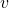of a trainseconds after leaving a station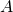for portion of the journey between two stationsand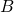,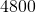ft. apart.

 Speed in feet per second.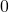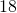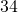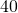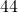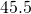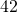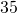Time in seconds.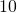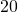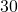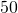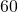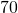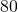Estimate the acceleration after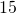seconds and the distance travelled in theseconds. If the train continues to move with the uniform speed offt. per second until the brakes are applied, producing a uniform retardation of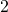ft. per sec. per sec., find the total time taken in going fromto.

##### Question 2

(a) A particle describes simple harmonic motion of amplitude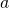, the period being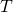. Prove that at distance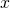from its mean position its speedis given by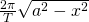.

(b) If the amplitude of the motion of bob of a simple pendulum isfoot and the period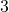seconds, find the time occupied by the bob in passing between points which are distance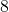” and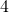” from the mean position and are on the same side of it.

##### Question 3

Prove that two bodies moving in different straight lines with uniform velocities will meet if their relative velocity is in the straight line joining them.

A ship steaming in a direction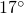North of East is seen by a submarine which is in a direction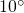East of South from the ship. Find in what direction a torpedo travelling atmiles per hour must be fired in order to strike ship.

##### Question 4

A bullet of massoz. travelling horizontally with a speed of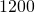ft. per second becomes embedded in a block of wood of masslb. suspended by a light steel wire, the C.G. of the block beingft. below the point of suspension. Find the vertical distance through which the block rises.

##### Question 5

When the motors of an electric train of effective weight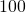tons are working at the rate ofH.P., a uniform speed ofmiles per hour is attained on the level. Assuming the frictional resistances to remain the same, what is the maximum speed attainable with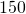H.P. when ascending an incline ofin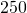?

##### Question 6

Show that the centre of gravity ofequal particles placed at the three vertices of a triangle respectively coincide with the centre of gravity of the area of the triangle. The parallel side of a trapezium are of lengthsand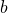and the perpendicular distance between them is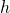. Find the distance of the C.G. from the side.

##### Question 7

What are the laws of solid friction?

A body of weight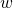is dragged along a horizontal plane, coefficient of friction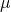, by a cord inclined at an angle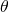to the horizontal; find the tension in the cord. For what value ofis the tension a minimum? A body is placed on a plane, coefficient of frictioninclined at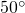to the horizontal; find the velocity acquired in one second.

##### Question 8

A triangular roof-truss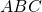has a horizontal span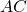of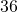feet and angleis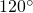,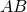and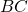being of equal length. The roof-truss is hinged atand simply supported on rollers at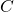(so that the supporting force atis vertical). It is loaded as follows: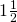tons perpendicular toat its mid-point at. Find the reactions of the supporting forces atandand the tensions in.

##### Question 9

A body of weightlb. is moving due East with a velocity of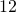ft. per second, and forty seconds later it is moving North-East with a velocity offt. per second. What is the change in velocity? What constant force acting during the interval would produce the change? Show that in the latter case the hodograph is a straight line.

##### Question 10

Assuming that the wheels of a motor-car are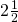feet in diameter, what is the angular velocity of a wheel when the car s travelling at the rate ofmiles per hour? What is the velocity of a point on the surface of tyre (a) when vertical above the centre, (b) when the radius from the point to the centre is inclined at an angle of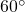to the upward vertical? What would be the force acting on a stud of massoz. embedded in the type when at its greatest height?

##### Citation:

State Examinations Commission (2018). State Examination Commission. Accessed at: https://www.examinations.ie/?l=en&mc=au&sc=ru

Malone, D and Murray, H. (2016). Archive of Maths State Exams Papers. Accessed at: http://archive.maths.nuim.ie/staff/dmalone/StateExamPapers/

##### Licence:

“Contains Irish Public Sector Information licensed under a Creative Commons Attribution 4.0 International (CC BY 4.0) licence”.

The EU Directive 2003/98/EC on the re-use of public sector information, its amendment EU Directive 2013/37/EC, its transposed Irish Statutory Instruments S.I. No. 279/2005, S.I No. 103/2008, and S.I. No. 525/2015, and related Circulars issued by the Department of Finance (Circular 32/05), and Department of Public Expenditure and Reform (Circular 16/15 and Circular 12/16).

Note. Circular 12/2016: Licence for Re-Use of Public Sector Information adopts CC-BY as the standard PSI licence, and notes that the open standard licence identified in this Circular supersedes PSI General Licence No: 2005/08/01.